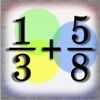# Fraction: Add, Compare, Subtract, Divide, Multiply, Reduce Fractions, LCM & GCD - By Verosocial Studio - Lumos Educational App Store51
Price -\$0.99
\$0.99

#### DESCRIPTION:

Featured by Apple under Ã¢â¬ËNew and Noteworthy' in the United States for iPhone and iPad > Productivity The Fraction+ calculator app is a tool to help you calculate fractions. Ã¢â¬Â¢ Fractions (Add) Ã¢â¬Â¢ Fractions (Compare) Ã¢â¬Â¢ Fractions (Divide) Ã¢â¬Â¢ Fractions (Multiply) Ã¢â¬Â¢ Fractions (Reduce) Ã¢â¬Â¢ Fractions (Subtract) Ã¢â¬Â¢ Greatest Common Factor Ã¢â¬Â¢ Least Common Multiple Also included is a commuter calculator to save money and time on your daily commute. The app consists of multiple calculators and

#### OVERVIEW:

Fraction: Add, Compare, Subtract, Divide, Multiply, Reduce Fractions, LCM & GCD - By Verosocial Studio is a free educational mobile app By Verosocial Studio.It helps students in grades 3 practice the following standards 3.NF.A.3.D.

This page not only allows students and teachers download Fraction: Add, Compare, Subtract, Divide, Multiply, Reduce Fractions, LCM & GCD - By Verosocial Studio but also find engaging Sample Questions, Videos, Pins, Worksheets, Books related to the following topics.

1. 3.NF.A.3.D : Compare two fractions with the same numerator or the same denominator, by reasoning about their size, Recognize that valid comparisons rely on the two fractions referring to the same whole. Record the results of comparisons with the symbols >, =, or <, and justify the conclusions, e.g., by using a visual fraction model. (Grade 3 expectations in this domain are limited to fractions with denominators 2, 3, 4, 6, and 8.).

3

#### STANDARDS:

3.NF.A.3.D

Developer: Verosocial Studio

Software Version: 7.4

Category: Education

### RELATED APPSEdSearch WebSearch